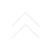#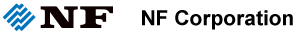Driving the future of technology : Original solution in measurement and control

## Lecture for Measurement

Posted 19/07/2019

### Voltage Amplifier ("Voltage-to-Voltage Amplifier")

#### 5. Selection of Preamplifier According to Signal-source Impedance

So how do we choose a preamplifier?

As a performance index of a preamplifier, an impedance value (ZOPTIMUM) obtained by the following formula is used.

ZOPTIMUM[Ω] = (Equivalent input noise voltage density [V/√Hz] ÷
(Equivalent input noise current density [A/√Hz])

ZOPTIMUM is a measure of the signal-source impedance at which the preamplifier can demonstrate low noise. As for a preamplifier, signal-source impedance below ZOPTIMUM is a desirable signal source.

ZOPTIMUM is calculated as follows: for SA-230F, it is given by 0.25 nV/√Hz÷5.0 pA/√Hz=50 Ω; for SA-220F5, it is given by 0.5 nV/√Hz÷200 fA/√Hz=2.5 kΩ.

According to the calculated value, a suitable preamplifier for a sensor is determined. Equivalent input noise voltage density (including the signal-source impedance of the preamplifier) is given by the following formula.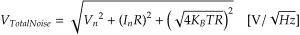In this equation, Vn is equivalent input noise voltage density [V/√Hz] of the amplifier itself, In is equivalent input noise current density [A/√Hz] of the amplifier itself, R is signal-source impedance. (output impedance of sensor, etc) [Ω], KB is Boltzmann’s constant [J/K], and T is absolute temperature [K].

By using this equation to simulate the output impedance of the sensor when one of three resistors with different resistances (0 Ω, 50 Ω, and 1 kΩ) is connected to the input, it is possible to determine whether SA-230F5 or SA-220F5 is classified as low noise.

Values of ZOPTIMUM and equivalent input noise voltage density when the resistors are connected are listed in Table 1. Also, thermal-noise values (signal source) at room temperature of the connected resistors are also listed.

With the 0 Ω resistor is connected (i.e., with the input shorted), SA-230F5 provides good low-noise performance. Connecting the 50 Ω resistor will make a difference between SA-230F5 and SA-220F5, albeit very small. However, when 1 kΩ resistor is connected, SA-220F5 attains lower noise than SA-230F5.

SA-230F5 uses a bipolar transistor as the input device. In the bipolar transistor, a base current is flowing when the transistor operates. Due to the base current, equivalent input noise current density also increases. Since the current is converted into a voltage when it flows through the resistor, the larger resistance, the greater effect of equivalent input noise current density.

On the contrary, the input of SA-2205F is connected to a junction field-effect transistor (junction FET or JFET). Only a very small amount of current flows in the gate of the FET; therefore, equivalent input noise current density stays at a small value.

As a result, when considering actual connections and operations of a sensor, the FET input often shows better noise characteristics than the bipolar-transistor input.

Table

Pattern ZOPTIMUM 0 Ω 50 Ω 1 kΩ
SA-230F5 50 Ω 0.25 nV/√Hz 0.98 nV/√Hz 6.45 nV/√Hz
SA-220F5 2.5 kΩ 0.50 nV/√Hz 1.04 nV/√Hz 4.11 nV/√Hz
Reference: Thermal noise of signal source N/A 0 nV/√Hz 0.91 nV/√Hz 4.07 nV/√Hz

ZOPTIMUM of the SA series is shown in Figure.

In some cases, ZOPTIMUM cannot be read from the data sheet of the preamplifier.

In such cases, as a guideline, it is recommended to select a preamplifier with input impedance more than 10 times the signal-source impedance. For example, if source impedance is 100 Ω, then, input impedance of 1 kΩ or more is recommended.

In the case a sensor with unknown signal-source impedance is to be used (along with a preamplifier that can be used for general purposes without limiting its application), it is recommended to select first a preamplifier that adopts an FET input.

Figure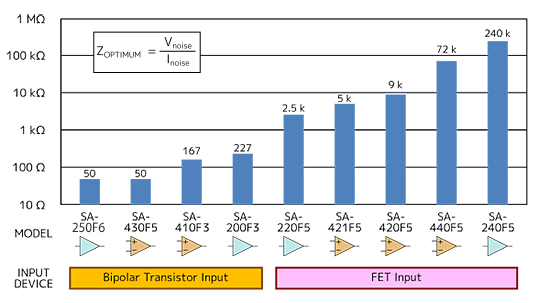Related Product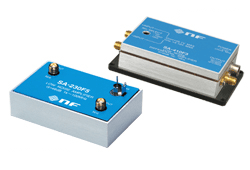Extremely low noise amplifier SA Series

Extremely low noise, excellent stability, wideband preamplifier for detection of extremely small signals

Low noise
• Adopted our exclusive circuit applying negative feedback technology
SA-230F5: at input impedance of 50 Ω, noise figure of 0.6 dB and equivalent input noise voltage density of 0.25 nV/√Hz
•  Wideband
• A wide frequency band from low-frequency signals including DC (SA-200F3/240F5/410F3/440F5) to high-frequency, high-speed signals (SA-220F5/230F5/430F5) exceeding 100 MHz is covered.
•  Input mode according to signal source
• Single-ended (single-wire-grounded) input, single-ended FET input, differential input, and differential FET input

Related keywords :  Ultra low noise amplifier, LNA

Recent post